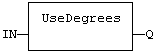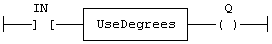# UseDegrees

Function - Sets the unit for angles in all trigonometric functions.

## Inputs

IN : BOOL     If TRUE, turn all trigonometric functions to use degrees
If FALSE, turn all trigonometric functions to use radians (default)

## Outputs

Q : BOOL      TRUE if functions use degrees before the call

## Remarks

This function sets the working unit for the following functions:

 SIN COS TAN ASIN ACOS ATAN ATAN2 sine cosine tangent arc-sine arc-cosine arc-tangent arc-tangent of Y / X

## ST Language

Q := UseDegrees (IN);

## FBD Language## LD Language

(* Input is the rung. The rung is the output *)Op1: LD   IN
UseDegrees
ST   Q